×

Search anything:

# Detect Cycle in Undirected Graph using Union Find algorithm

#### Algorithms Graph AlgorithmsGet this book -> Problems on Array: For Interviews and Competitive Programming

In this article, we've explored the approach to detect a cycle in undirected graph using union-find algorithm. This takes O(VE) Time Complexity.

1)Glance of Union-Find algorithm
2)Introduction
3)Algorithm to find cycle using Union-Find
4)Understanding algorithm through example
5)Implementation in Python
6)Conclusion

## 1)Glance of Union-Find algorithm

Disjoint-Set is the data structure used in case of Union-Find algorithm.

Disjoint-Set is defined as follows:

Given a set of elements. These items are partitioned into several subsets such that each element is restricted to a particular subset.

In simple terms, A Disjoint-Set data structure is a group of sets where no two sets contain the same element.

It is also called union-find data structure because it supports Union and Find operations on subsets.Let's understand those terms..

Find: Each subset is represented by an element of that subset known as the set-representative.This operation returns the set-representative of a particular element to which it belongs to. This operation has worst case time complexity of O(N) where N is the number of elements in the set since it finds the set-representative recursively.

Union:In this operation two subsets are merged into one subset.The set-representative of the merged subset will one among the set-representatives of those subsets which are merged. This operation has time complexity of O(1).

There is one more operation called make-set operation.

Make-Set:This operation creates disjoint sets for each element in the given set.Intially set-representative for each disjoint set will be the element contained in it. This operation has time complexity of O(1).

Here each subset can be called as a disjoint-set.

## 2)Introduction

In simple terms, a graph is said to have cycle if there exists a path whose start vertex and end vertex are the same.It is termed as cyclic graph.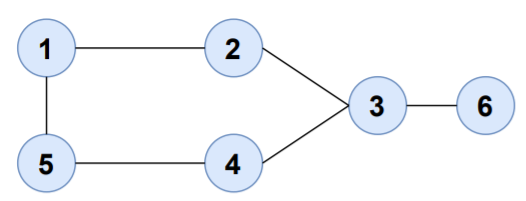Consider the path,

1-2-3-4-5-1

The start and end vertices are the same.Hence, the graph contains cycle.

## 3)Algorithm to find cycle using Union-Find

1)Create disjoint-sets for each of the vertices in the graph.
2)Repeat the following steps for each edge(u,v) in the graph
-If u and v both belong to the same disjoint-set then cycle exists in the graph.
-Else merge the disjoint-sets in which u and v are present.

## 4)Understanding algorithm through example

Let's use the Union-Find algorithm to find cycle in an undirected graph.

Find the edges from the graph.

1-2
2-3
3-4
3-6
4-5
1-5

As there are six vertices, we'll create six disjoint-sets.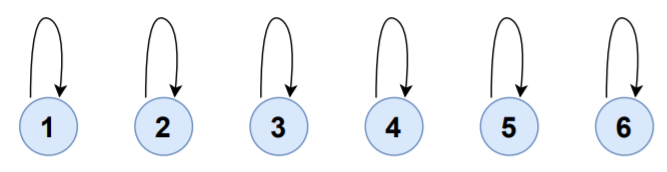SET ELEMENTS SET-REPRESENTATIVE
S1 {1} 1
S2 {2} 2
S3 {3} 3
S4 {4} 4
S5 {5} 5
S6 {6} 6

Consider the edge 1-2,

FIND(1)=1
FIND(2)=2

UNION(1,2)

S1,S2 will be merged to S1 and set-representative of S1 will now be 1.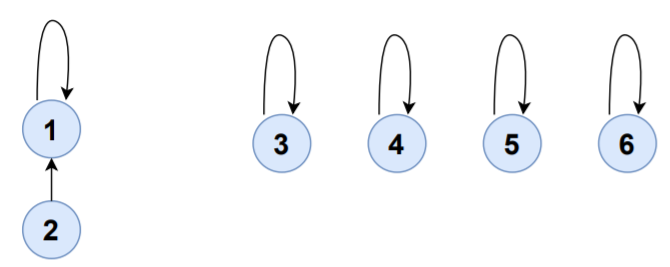SET ELEMENTS SET-REPRESENTATIVE
S1 {1,2} 1
S3 {3} 3
S4 {4} 4
S5 {5} 5
S6 {6} 6

Consider the edge 2-3,

FIND(2)=1
FIND(3)=3

UNION(1,3)

S1,S3 will be merged to S1 and set-representative of S1 will now be 1.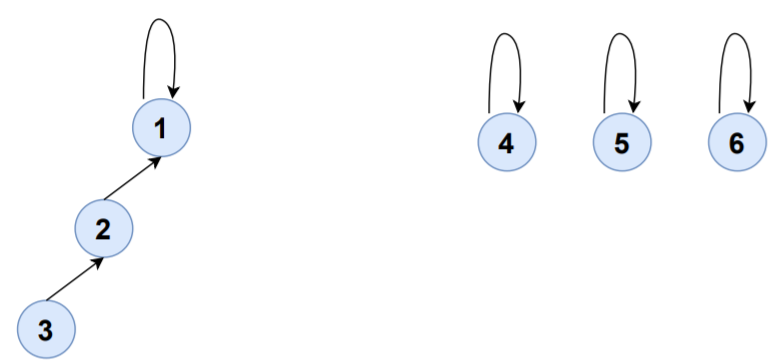SET ELEMENTS SET-REPRESENTATIVE
S1 {1,2,3} 1
S4 {4} 4
S5 {5} 5
S6 {6} 6

Consider the edge 3-4,

FIND(3)=1
FIND(4)=4

UNION(1,4)

S1,S4 will be merged to S1 and set-representative of S1 will now be 1.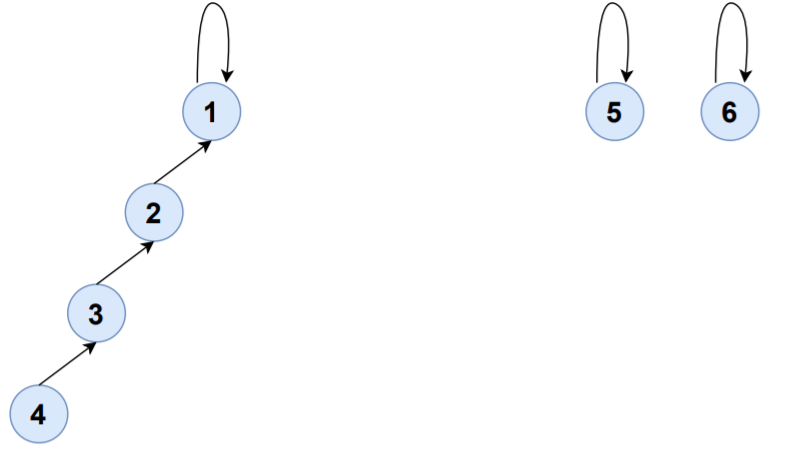SET ELEMENTS SET-REPRESENTATIVE
S1 {1,2,3,4} 1
S5 {5} 5
S6 {6} 6

Consider the edge 3-6,

FIND(3)=1
FIND(6)=6

UNION(1,6)

S1,S6 will be merged to S1 and set-representative of S1 will now be 1.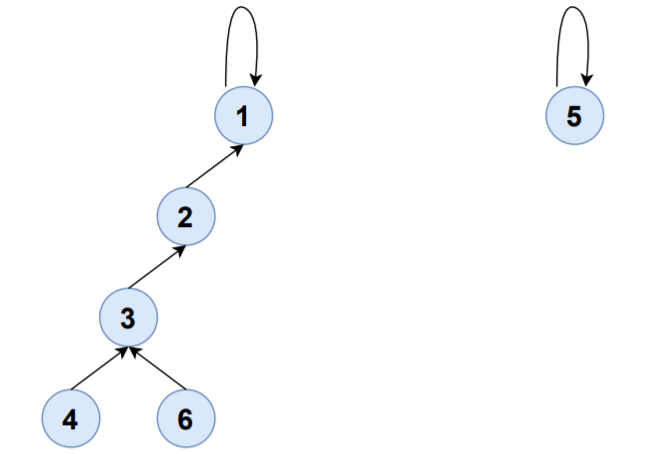SET ELEMENTS SET-REPRESENTATIVE
S1 {1,2,3,4,6} 1
S5 {5} 5

Consider the edge 4-5,

FIND(4)=1
FIND(5)=5

CALL UNION(1,5)

S1,S5 will be merged to S1 and set-representative of S1 will now be 1.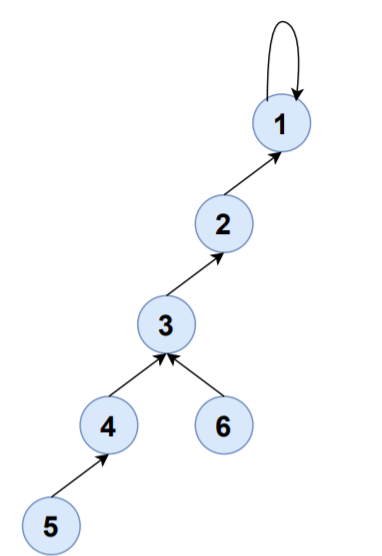SET ELEMENTS SET-REPRESENTATIVE
S1 {1,2,3,4,5,6} 1

Consider the edge 1-5,

FIND(1)=1
FIND(5)=1

Here FIND(1)=FIND(5) that means both belong to same disjoint set. Hence,the graph has a cycle.

## 5)Implementation in Python

``````class Union_Find:
def make_set(self,n):
self.disjoint_set={vertex:vertex for vertex in range(1,n+1)}#Initializing n subsets and make contained vertex itself the set-representative for each subset
def find(self,vertex):
if self.disjoint_set[vertex]==vertex:#A set-representative can be found if v:v
return vertex
return self.find(self.disjoint_set[vertex])
def union(self,x,y):
set_representative_X=self.find(x)#find set-representative of x
set_representative_Y=self.find(y)#find set-representative of y
self.disjoint_set[set_representative_Y]=set_representative_X#make set-representative of y as x

def isCyclic(edges,no_of_vertices):
ds=Union_Find()
ds.make_set(no_of_vertices)
for x,y in edges:
if ds.find(x)==ds.find(y):#if set-representatives of both the vertices are the same then cycle exists
print("Cycle exists in the graph!")
return
ds.union(x,y)#merge subsets x and y
print("Cycle doesn't exist in the graph!")
V=int(input())
E=int(input())
edges=[]
for t in range(E):
x,y=[int(x) for x in input().split()]
edges.append([x,y])
isCyclic(edges,V)
``````

Input:

``````V=6
E=6
edges=[[1,2],[2,3],[3,4],[3,6],[4,5],[1,5]]
``````

Output:

``````Cycle exists in the graph!
``````

Consider the graph which does not contain a cycle..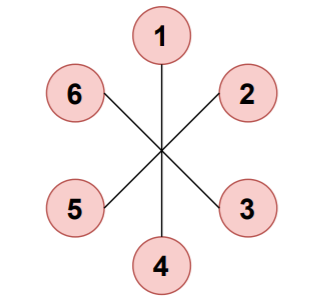Input:

``````V=6
E=6
edges=[[1,4],[2,5],[3,6]]
``````

Output:

``````Cycle doesn't exist in the graph!
``````

## 6)Conclusion

The time complexity of Union-Find algorithm is O(V), where V is the number of vertices in the graph, in the worst case .

As we need to visit each edge it takes time of O(E) where E is the number of edges in the graph.

Hence, the overall time complexity of detecting cycle in Undirected Graph using Union-Find is O(VE).

In this case, V is number of vertices and E is number of edges. In case of a dense graph, E is of the order of O(V2). So, in a dense graph, this approach takes O(V3) Time Complexity.

With this article at OpenGenus, you must have the complete idea of how to Detect Cycle in Undirected Graph using Union Find algorithm.